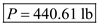# Two 8° wedges of negligible weight are used to move and position and block position a 530-Ib block Knowing that the coefficient of static friction is 0.40 at all surfaces of contact, determine the magnitude of the force P for which motion of the block is impending.

Question-AnswerCategory: Engineering MechanicsTwo 8° wedges of negligible weight are used to move and position and block position a 530-Ib block Knowing that the coefficient of static friction is 0.40 at all surfaces of contact, determine the magnitude of the force P for which motion of the block is impending.

Two 8° wedges of negligible weight are used to move and position and block position a 530-Ib block  Knowing that the coefficient of static friction is 0.40 at all surfaces of contact, determine the magnitude of the force P for which motion of the block is impending.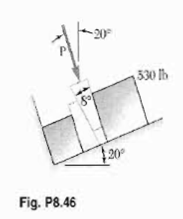Step: 1

Free body diagram for block:Step: 2

Given:
Coefficient of static friction,From equilibrium equations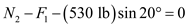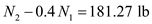…… (1)

Step: 3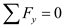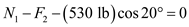…… (2)

Step: 4

So, by solving (1) and (2) we getand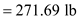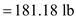Step: 5

Free body diagram for wedge:Step: 6

From equilibrium equation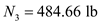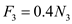Step: 7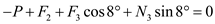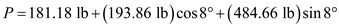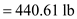The magnitude of force is